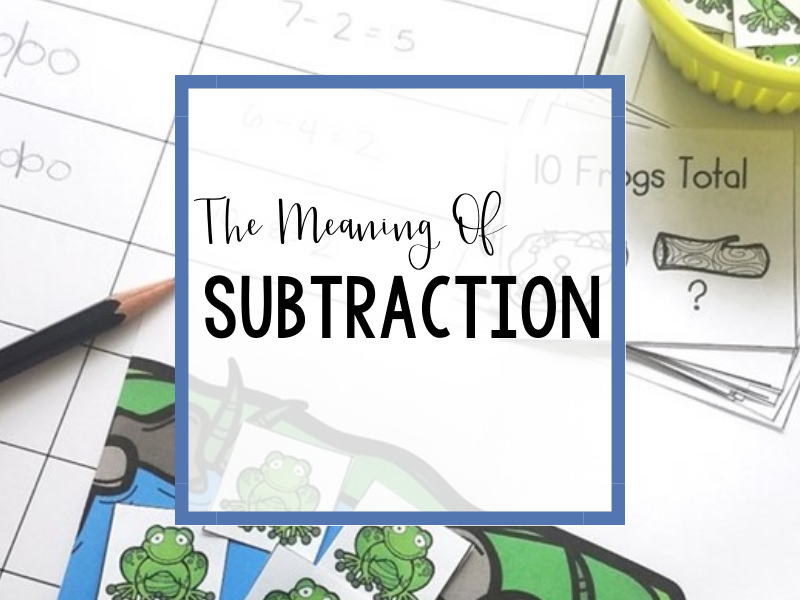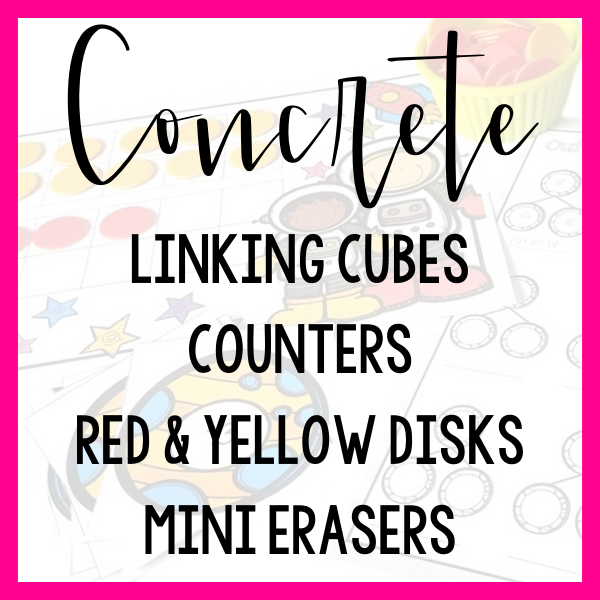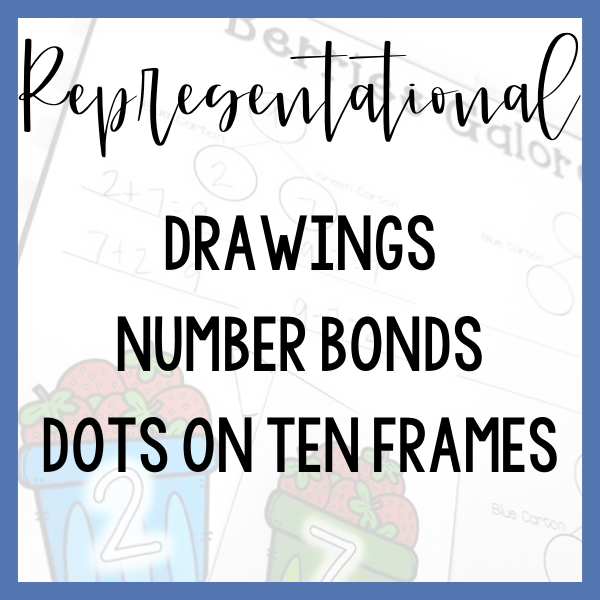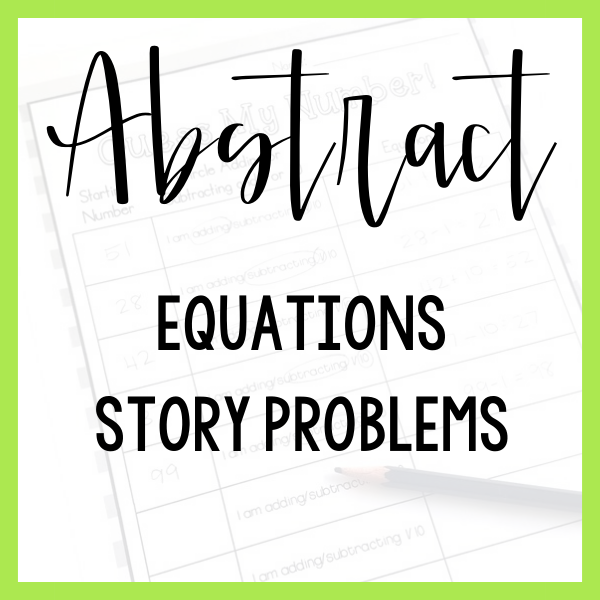# Let's Get Started

Students need to know more than the mechanics or procedures for how to subtract. If they are to generalize this skill to other settings and utilize subtraction in a variety of applications they need to understand what subtraction is, what the symbols mean, and when to apply the skill.The 5 examples listed are certainly not an exhaustive list of tools and lessons that could be used to teach this skill.

They are rather a sample progression from hands-on to abstract thinking!

Which step represents your students’ current level of understanding?

Subtraction, ultimately, means finding the difference between two numbers. To your students, this ultimately looks like a comparison, taking away or taking apart. In this introduction we are focusing on subtraction as meaning taking apart.

Consider introducing the meaning of subtraction without numbers at all! Taking apart an ice cream cone yields a “scoop” and a “cone”. Your students can practice putting these pictures into number bonds and equations to solidify their understanding of subtraction and the minus sign as meaning “take apart”.

It is critical to use math vocabulary including the words “part” and “whole” as your students begin to understand subtraction.

Together with your students, explore a set of 5 linking cubes. How many different ways can the 5 cubes be broken into two parts?

Record your students findings in number bonds and as equations. This will help your students to understand subtraction in hands-on, representational and written forms.

Increase complexity and decrease overgeneralization by extending exploration outside of the number 5.

Provide your students with sets of 1, 2, 3, 4, or 5 linking cubes and ask them to explore to find all of the ways they can decompose these numbers.

As they explore, put the blocks into a number bond to show how the whole was broken into parts. Create a written number bond that shows the same information along with an equation to match.

Continue to link hands-on, number bonds and equations whenever possible to help your students see connections between models.

Provide your students with a variety of hands-on materials such as linking cubes or mini erasers and ask them to solve simple word problems with a take apart context.

As your students explore the context of the story problem, model the action of the problem in number bonds and record an equation that matches the context.

Continue to emphasis the language of “part” and “whole” as you take numbers apart.

If you take away the hands-on support, are your students still solid in their understanding of subtraction?

Continue to explore word problems. As you do, ask your students to model with math materials such as linking cubes or mini erasers.

Ask students to draw a picture that matches their math materials. This will support the transition from concrete to representational.

Finally, ask your students to write an equation that matches their model. When your students see subtraction equations in the future, they will make connections between the hands-on work, drawings and those equations. Rather than a set of arbitrary symbols, subtraction will have meanign for your students!

# Tools To USe